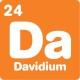# What's new## Divide by this

, 10 August 2023

Difficulty: Extreme
A teacher wrote a whole number on the whiteboard and asked her students to say what numbers it could divide by.

The 1st student said, “the number is divisible by 1.”

The 2nd student said, “the number is divisible by 2.”

The 3rd student said, “the number is divisible by 3.”

And this continued until the 7th student said, “the number is divisible by 7.”

The teacher said only two students spoke incorrectly and these students spoke one after the other (consecutively).

What are the two incorrect numbers?

Scroll down or click for a hint, or the answer!## Brainteaser hint

The two students who spoke incorrectly were one after the other – so one of them said an odd number and the other said an even one.

For a bigger hint:

If a number is divisible by 2 and also by 3, then it will be divisible by 6 (because 2 x 3 = 6).This brainteaser is really tricky, so it’s okay to get a bit stuck.

We know that the 2 incorrect numbers are consecutive. We can start by writing down all the pairs of consecutive numbers:

(1,2) (2,3) (3,4) (4,5) (5,6) (6,7)

And try to eliminate them.

Every whole number is divisible by 1, so 1 can’t be incorrect. We can eliminate (1,2).

If 2 is incorrect, the number on the board is odd. And that means 4 and 6 are also incorrect (odds aren’t divisible by even numbers). That’s 3 incorrect answers, which breaks the rules, because there are only supposed to be 2 incorrect answers. We can eliminate (2,3) since 2 must be correct.

If 3 is incorrect, then there’s no way the number can be divisible by 6. That breaks the rules, because the incorrect answers are supposed to be consecutive (3 and 6 are not consecutive). We can eliminate (3,4) since 3 must be correct.

Since both 2 and 3 are correct, the number on the board will also be divisible by 6 (because 2 x 3 = 6). This means 6 is also a correct answer we can rule out.

Now, we have ruled out 1, 2, 3 and 6. The only consecutive pair left is 4 and 5. So that’s our answer!

For a final check, let’s try and make up a number that could go on the board.

To start with, 6 is a natural choice. It is divisible by 1, 2, 3 and 6, and it’s not divisible by 4 or 5. Unfortunately, it’s also not divisible by 7.

What’s an easy number that’s divisible by 6 and 7? 6 x 7 = 42.

If we divide it out we get:

42 ÷ 1 = 42
42 ÷ 2 = 21
42 ÷ 3 = 14
42 ÷ 4 = 10.5
42 ÷ 5 = 8.4
42 ÷ 6 = 7
42 ÷ 7 = 6

It worked! 4 and 5 must be the two incorrect numbers.

Categories:

## Similar posts

1.Big clue in the question is that it’s likely to be a factor of 7, as unlikely to have continued to 7 if 7 was incorrect. Lowest factor of 7 and 3 is 21, then to be a factor of 2 and 6 double it for 42. This leaves 4 and 5 as the incorrect numbers.

1.Hi Peter,
Nice spotting! There’s a trickier version of this puzzle that has 31 people in the class – I think the number on the board ends up being a lot bigger than 42…

2.That was a trickier puzzle. My calculator doesn’t seem to have enough digits, though 6370744980600 seems like a solution, a lot bigger than 42. I guessed one number would be prime, and the other number likely to be 16, explaining why it’s to 31 (one less than 32, or 2×16). So guessing 16 and 17 as the pair then trial and error low factors of the other numbers.

3.looks pretty good to me! you might have one extra power of 3 in there, but that certainly doesn’t make your answer wrong.